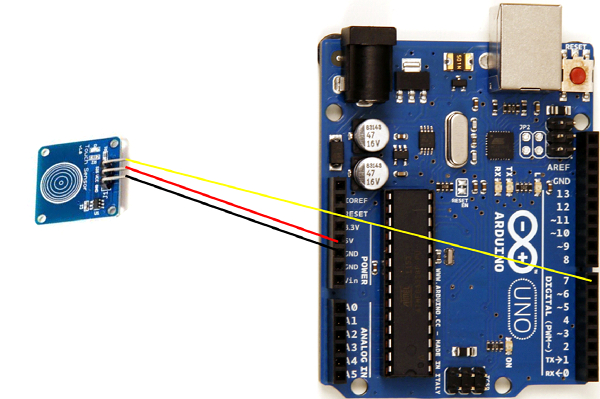# Interface touch sensor with Arduino

A touch sensor looks like the one below −It has 3 pins − Vcc, GND and Signal. Whenever someone touches the sensor, the signal pin goes HIGH (it generally outputs LOW when not touched). Thus, we just have to digitalRead the Signal Pin and determine if the sensor is being touched.

### Circuit Diagram

The circuit diagram is quite straightforward as shown belowAs you can see, the GND pin of touch sensor is connected to GND pin of Arduino, the Vcc pin to 5V and the SIG pin to pin 7 of the Arduino.

### Example Code

The code is also quite straightforward, as you can see below −

int signalPin = 7;
void setup() {
// put your setup code here, to run once:
Serial.begin(9600);
pinMode(signalPin,INPUT);
}
void loop() {
// put your main code here, to run repeatedly:
}